# Computation of an infinite integral using a dynamical extrapolation method

Let us consider the integral
with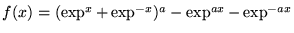and
.

This integral arises in a study of the total electronic energy of crystals using the tight binding approximation . The evaluation of
was a problem posed in .
is computed using Romberg's integration method with the CADNA library.

A theorem like those given in  has been established for the dynamical control of Romberg's method . Combining it with two similar theorems, we can estimate the number of exact significant digits of the result obtained, which are common with the exact value of the infinite integral (including both the truncation error and the round-off error).

To combine efficiency and reliability the same expression ofis not used at both bounds of the interval. One worry is to avoid cancellations at the infinite bound of the interval.

Thereforeis computed as,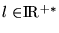,

where
and, withFunctionsandare mathematically equivalent, but not numerically !
, with
.
is approximated by an iterate of the sequence
.

How to choose the optimal iterate? Iterations are performed until the difference between two successive iterates is not significant. Using the CADNA library, the stopping criterion is.

Computations stop ifandare stochastically equal, i.e. if the transformation ofintois only due to round-off errors. Further iterations are useless.

We have shown that if, for all, the exact significant bits of the optimal iterateare those of, up to one.is approximated by computing integrals on intervals of length.

Each of these integrals is computed using Romberg's method.

The approximation of an integral with Romberg's method is an iterate of a sequence which converges with an exponential speed to the exact value of this integral.

How to choose the optimal iterate ?

Approximations are computed until the difference between two successive iterates is not significant.

We have shown that, for each integral, the approximation obtained has its exact significant bits common to the exact value of the integral, up to one.
with
How to choose the optimal iterate
? Iterations are performed until the difference between two successive iterates is not significant.

Finally we have shown that using the CADNA library and the stopping criteria previously described, we can estimate the number of exact significant digits of the approximation ofobtained, which are common with the exact value of the integral.

## References

1
J.-M. Chesneaux, F. Jézéquel, Dynamical control of computations using the trapezoidal and Simpson's rules, Journal of Universal Computer Science, Vol. 4 (1), pp. 2-10, 1998.

2
W. Harrison, Total energies in the tight-binding theory, Phys. Rev., B23 (1981), pp. 5230-5245

3
F. Jézéquel, Dynamical control of computations using approximation methods, 16th IMACS world congress on Scientific Computation, Applied Mathematics and Simulation, Lausanne (Switzerland), August 2000.

4
Problem 95-7, SIAM Review, V 38, N 2, pp.324, 1996.

To get the C source code.

To get the FORTRAN source code.